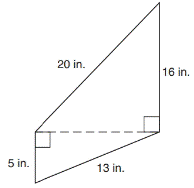Chapter 8.1, Problem 17EElementary Geometry For College St...

7th Edition
Alexander + 2 others
ISBN: 9781337614085

Solutions

Chapter
SectionElementary Geometry For College St...

7th Edition
Alexander + 2 others
ISBN: 9781337614085
Textbook Problem

In Exercises 9 to 18, find the areas of the figures shown or described.To determine

To find:

The area of the figure.

Explanation

1) Formula:

Finding the area of the figure is followed by identifying the figure and find area with the suitable geometry formulae,

Area of the right triangle is given by,

A=12(ab) unit2

Where a and b are the length of the sides.

2) Calculation:

Given,

Here, the give figure is a compound figure of two right triangle.

Thus,

Area of given figure=area of right triangle(A1)+area of right triangle(A2)

From the right ABD

AB=13 in, AD=5 in

By pythagorean theorem,

(5)2+(DB)2=(13)2

25+(DB)2=169

Add (25) on both sides,

25+(DB)2+(25)=169+(25)

(DB)2+2525=16925

(DB)2=144

Still sussing out bartleby?

Check out a sample textbook solution.

See a sample solution

The Solution to Your Study Problems

Bartleby provides explanations to thousands of textbook problems written by our experts, many with advanced degrees!

Get Started

In Exercises 41-48, find the indicated limit given that limxaf(x)=3 and limxag(x)=4 42. limxa2f(x)

Applied Calculus for the Managerial, Life, and Social Sciences: A Brief Approach

Find f. f(t) = 2t 3 sin t, f(0) = 5

Single Variable Calculus: Early Transcendentals, Volume I

Convert the expressions in Exercises 6584 to power form. 25x3

Finite Mathematics and Applied Calculus (MindTap Course List)

An integral for the solid obtained by rotating the region at the right about the x-axis is:

Study Guide for Stewart's Single Variable Calculus: Early Transcendentals, 8th